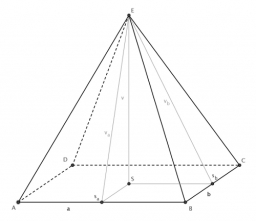# School model

The beech school model of a regular quadrilateral pyramid has a base 20 cm long and 24 cm high. Calculate a) the surface of the pyramid in square decimeters, b) the mass of the pyramid in kilograms if the density of the beech is ρ = 0,8 g/cm ^ 3

S =  14.4 dm2
m =  2.56 kg

### Step-by-step explanation:Did you find an error or inaccuracy? Feel free to write us. Thank you!Tips to related online calculators
Tip: Our volume units converter will help you with the conversion of volume units.
Pythagorean theorem is the base for the right triangle calculator.

#### You need to know the following knowledge to solve this word math problem:

We encourage you to watch this tutorial video on this math problem:

## Related math problems and questions:A quadrilateral pyramid, which has a rectangular base with dimensions of 24 cm, 13 cm. The height of the pyramid is 18cm. Calculate 1/the area of the base 2/casing area 3/pyramid surface 4/volume of the pyramidFind the volume and surface of a regular quadrilateral pyramid if the bottom edge is 45 cm long and the pyramid height is 7 cm.
• Tetrahedral pyramidCalculate the regular tetrahedral pyramid's volume and surface if the content area of the base is 20 cm2, and the deviation angle of the side edges from the plane of the base is 60 degrees.What is the volume of a regular quadrilateral pyramid if its surface is 576 cm2 and the base edge is 16 cm?We have a regular quadrilateral pyramid with a base edge a = 10 cm and a height v = 7 cm. Calculate 1/base content 2/casing content 3/pyramid surface 4/volume of the pyramidWhat is the volume of a regular quadrilateral pyramid if the edge of the base is 8 cm long and the height of the side wall is 5 cm?
• 4side pyramidCalculate the volume and surface of 4 sides regular pyramid whose base edge is 4 cm long. The angle from the plane of the sidewall and base plane is 60 degrees.
• Pentagonal pyramidThe height of a regular pentagonal pyramid is as long as the edge of the base, 20 cm. Calculate the volume and surface area of the pyramid.
• Pyramid 4sidesCalculate the volume and the surface of a regular quadrangular pyramid when the edge of the base is 4 cm long and the height of the pyramid is 7 cm.The quadrilateral pyramid has a rectangular base of 24 cm x 3.2dm and a body height of 0.4m. Calculate its volume and surface area.In a regular quadrilateral pyramid, the height is 6.5 cm and the angle between the base and the side wall is 42°. Calculate the surface area and volume of the body. Round calculations to 1 decimal place.Find the surface area of a regular quadrilateral pyramid which has a volume of 24 dm3 and a height of 45 cm.The regular quadrilateral pyramid has a volume of 24 dm3 and a height of 45 cm. Calculate its surface.Find the height and surface of a regular quadrilateral pyramid with a base edge a = 8cm and a wall height w = 10cm. Sketch a picture.A regular quadrilateral pyramid has a volume of 24 dm3 and a base edge a = 4 dm. Calculate: a/height of the pyramid b/sidewall height c/surface of the pyramidA regular tetrahedral pyramid is given. Base edge length a = 6.5 cm, side edge s = 7.5 cm. Calculate the volume and the area of its face (side area).The concrete pedestal in the shape of a regular quadrilateral truncated pyramid has a height of 12 cm, the pedestal edges have lengths of 2.4 and 1.6 dm. Calculate the surface of the base.